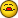If with countif or counta

J

jamalhakem

Hi
Any help?
I want to count cells that contain numbers with criteria, I used these
functions, but it returns false, the range can contain data or are
blank, I want to count the nonblank only that meet the criteria <50 or
<60. What I wanted is to count if the cells contain numbers, and not
count the blank cells, and if all cells are empty, return blank””.
30 50 60 50 70 80 60 20 50 60 60 80 90

IF(ISNUMBER(D11:F11);COUNTIF(D11:F11;"<50")
+IF(ISNUMBER(G11:J12);COUNTIF(G11:J11;"<60")
+IF(ISNUMBER(K11:L11);COUNTIF(K11:L11;"<50")
+IF(ISNUMBER(M1111);COUNTIF(M1111;"<60")
+IF(ISNUMBER(Q11:S11);COUNTIF(Q11:S11;"<50")
+IF(ISNUMBER(T11:W11);COUNTIF(T11:W11;"<60")
+IF(ISNUMBER(X11:Y11);COUNTIF(X11:Y11;"<50");"")))))))
Jam

T

T. Valko

--
Biff
Microsoft Excel MVP

Hi
Any help?
I want to count cells that contain numbers with criteria, I used these
functions, but it returns false, the range can contain data or are
blank, I want to count the nonblank only that meet the criteria <50 or
<60. What I wanted is to count if the cells contain numbers, and not
count the blank cells, and if all cells are empty, return blank””.
30 50 60 50 70 80 60 20 50 60 60 80 90

IF(ISNUMBER(D11:F11);COUNTIF(D11:F11;"<50")
+IF(ISNUMBER(G11:J12);COUNTIF(G11:J11;"<60")
+IF(ISNUMBER(K11:L11);COUNTIF(K11:L11;"<50")
+IF(ISNUMBER(M1111);COUNTIF(M1111;"<60")
+IF(ISNUMBER(Q11:S11);COUNTIF(Q11:S11;"<50")
+IF(ISNUMBER(T11:W11);COUNTIF(T11:W11;"<60")
+IF(ISNUMBER(X11:Y11);COUNTIF(X11:Y11;"<50");"")))))))
Jam

R

Ron Rosenfeld

Hi
Any help?
I want to count cells that contain numbers with criteria, I used these
functions, but it returns false, the range can contain data or are
blank, I want to count the nonblank only that meet the criteria <50 or
<60. What I wanted is to count if the cells contain numbers, and not
count the blank cells, and if all cells are empty, return blank””.
30 50 60 50 70 80 60 20 50 60 60 80 90

IF(ISNUMBER(D11:F11);COUNTIF(D11:F11;"<50")
+IF(ISNUMBER(G11:J12);COUNTIF(G11:J11;"<60")
+IF(ISNUMBER(K11:L11);COUNTIF(K11:L11;"<50")
+IF(ISNUMBER(M1111);COUNTIF(M1111;"<60")
+IF(ISNUMBER(Q11:S11);COUNTIF(Q11:S11;"<50")
+IF(ISNUMBER(T11:W11);COUNTIF(T11:W11;"<60")
+IF(ISNUMBER(X11:Y11);COUNTIF(X11:Y11;"<50");"")))))))
Jam

At least in XL2007, COUNTIF with a number criteria ignores blanks (and text).

So an equivalent formula to count the values that meet your criteria would be:

=COUNTIF(D11:F11;"<50")
+COUNTIF(G11:J11;"<60")
+COUNTIF(K11:L11;"<50")
+COUNTIF(M1111;"<60")
+COUNTIF(Q11:S11;"<50")
+COUNTIF(T11:W11;"<60")
+COUNTIF(X11:Y11;"<50")

To add to that your criteria of returning a blank if all cells are empty, then
use this:

=IF(COUNT(D11:Y11)=0;"";
COUNTIF(D11:F11;"<50")
+COUNTIF(G11:J11;"<60")
+COUNTIF(K11:L11;"<50")
+COUNTIF(M1111;"<60")
+COUNTIF(Q11:S11;"<50")
+COUNTIF(T11:W11;"<60")
+COUNTIF(X11:Y11;"<50"))

--ron

=IF(COUNT(D11:Y11),SUM(COUNTIF(INDIRECT({"D11:F11","K11:L11","Q11:S11","X11:Y11"}),"<50"),COUNTIF(INDIRECT({"G11:J11","M1111","T11:W11"}),"<60")),"")